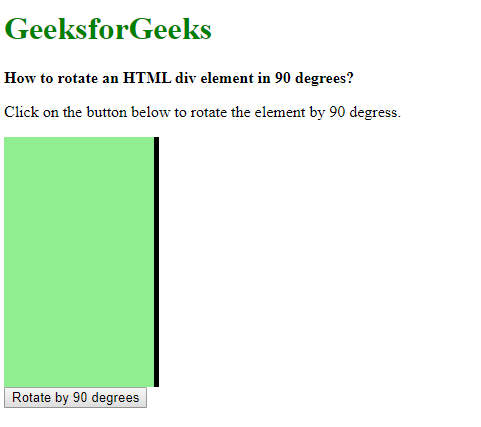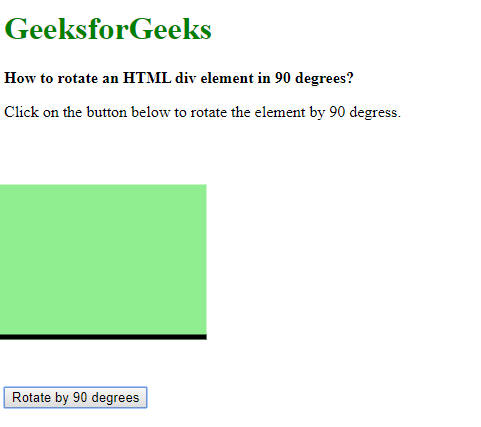# How to rotate an HTML div element 90 degrees using JavaScript ?

An element can be rotated 90 degrees by using the transform property. This property is used to move, rotate, scale and others to perform various kinds of transformation to elements.
The rotate() transformation function can be used as the value to rotate the element. It takes one parameter which defines the rotation angle. The rotation angle consists of two parts, the value of the rotation followed by the unit of rotation. The unit can be defined in degrees (deg), gradient (grad), radians (rad) and turns.

To rotate by 90 degrees any of the units can be used with their corresponding values. 90 degrees would equal to 100 gradient or 0.25 turns.

Applying this property to the required element would rotate it by 90 degrees.

Syntax:

```// Using CSS
transform: rotate(90deg);

// Using JavaScript
element.style.transform = 'rotate(90deg)';
```

Example: The example uses a rectangle and one side of a border to explain the rotation.

 ` ` `<``html``> ` ` `  `<``head``> ` `    ``<``title``> ` `        ``How to rotate an HTML div element ` `        ``90 degrees using JavaScript ? ` `    `` ` `     `  `    ``<``style``> ` `        ``.box { ` `            ``height: 250px; ` `            ``width: 150px; ` `            ``border-right: 5px solid; ` `            ``background-color: lightgreen; ` `        ``} ` `    `` ` ` ` ` `  `<``body``> ` `    ``<``h1` `style``=``"color: green"``> ` `        ``GeeksforGeeks ` `    `` ` `     `  `    ``<``b``> ` `        ``How to rotate an HTML div ` `        ``element in 90 degrees? ` `    `` ` `     `  `    ``<``p``> ` `        ``Click on the button below to rotate ` `        ``the element by 90 degress. ` `    `` ` `     `  `    ``<``div` `class``=``"box"``> ` `     `  `    ``<``button` `onclick``=``"rotateElem()"``> ` `        ``Rotate by 90 degrees ` `    `` ` `     `  `    ``<``script` `type``=``"text/javascript"``> ` `        ``function rotateElem() { ` `            ``document.querySelector('.box').style.transform ` `                       ``= 'rotate(90deg)'; ` `        ``} ` `    `` ` ` ` ` `  ` `

Output:

• Before clicking the button:• After clicking the button:My Personal Notes arrow_drop_upCheck out this Author's contributed articles.

If you like GeeksforGeeks and would like to contribute, you can also write an article using contribute.geeksforgeeks.org or mail your article to contribute@geeksforgeeks.org. See your article appearing on the GeeksforGeeks main page and help other Geeks.

Please Improve this article if you find anything incorrect by clicking on the "Improve Article" button below.